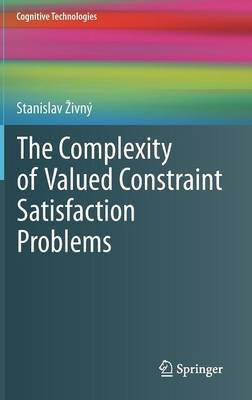•The Complexity of Valued Constraint Satisfaction Problems - Cognitive Technologies (Hardback)

(author)
£99.99
Hardback 170 Pages / Published: 20/10/2012
• We can order this

Usually dispatched within 3 weeks

The topic of this book is the following optimisation problem: given a set of discrete variables and a set of functions, each depending on a subset of the variables, minimise the sum of the functions over all variables. This fundamental research problem has been studied within several different contexts of discrete mathematics, computer science and artificial intelligence under different names: Min-Sum problems, MAP inference in Markov random fields (MRFs) and conditional random fields (CRFs), Gibbs energy minimisation, valued constraint satisfaction problems (VCSPs), and, for two-state variables, pseudo-Boolean optimisation.

In this book the author presents general techniques for analysing the structure of such functions and the computational complexity of the minimisation problem, and he gives a comprehensive list of tractable cases. Moreover, he demonstrates that the so-called algebraic approach to VCSPs can be used not only for the search for tractable VCSPs, but also for other questions such as finding the boundaries to the applicability of certain algorithmic techniques.

The book is suitable for researchers interested in methods and results from the area of constraint programming and discrete optimisation.

Publisher: Springer-Verlag Berlin and Heidelberg GmbH & Co. KG
ISBN: 9783642339738
Number of pages: 170
Weight: 453 g
Dimensions: 235 x 155 x 12 mm
Edition: 2012 ed.

MEDIA REVIEWS

From the reviews:

"The book is concerned with valued constraint satisfaction problems with finite domains, i. e., the problem of minimising the sum of functions that each depend on some subset of a finite set of discrete variables with finite domains. ... In all places the description is very precise and exact, detailed where needed. This makes it a very useful reference for researchers working in the field." (Thomas Jansen, zbMATH, Vol. 1283, 2014)